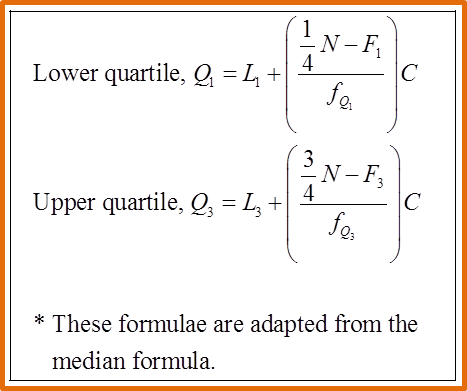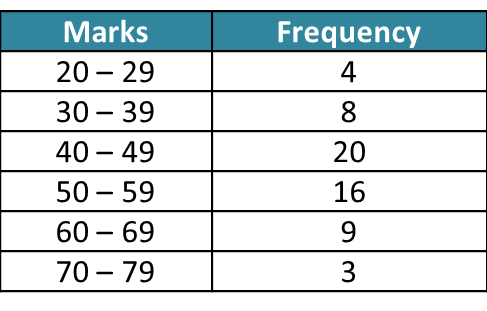# 7.2b Interquartile Range 2

Measures of Dispersion (Part 2)
7.2b Interquartile Range 2

(C) Interquartile Range of Grouped Data (with Class Interval)

The interquartile range of grouped data can be determined by Method 1 (using a cumulative frequency table) or Method 2 (using an ogive).Example:
The table below shows the marks obtained by a group of Form 4 students in school mathematics test.Estimate the interquartile range.

Solution:
Method 1: From Cumulative Frequency Table
Step 1:
Lower quartile, Q1 = the   $\frac{1}{4}{\left(60\right)}^{\text{th}}$ observation
= the 15thobservation

Upper quartile, Q3 = the   $\frac{3}{4}{\left(60\right)}^{\text{th}}$ observation
= the 45thobservationStep 2:

Step 3:

Step 4:
Interquartile Range
= upper quartile – lower quartile
= Q3Q1
= 57.625 – 41
= 16.625